# RD Sharma Solutions for Class 10 Maths Chapter 4 Triangles Exercise 4.2

Similar triangles and their properties are the prime focus in this exercise. Basic results on proportionality and theorems are examined in the exercise questions. The RD Sharma Solutions Class 10 can be used by the students to clarify their doubts and as a preparation tool for exams. The RD Sharma Solutions for Class 10 Maths Chapter 4 Triangles Exercise 4.2 PDF provided below can also be utilised by the students.

## RD Sharma Solutions for Class 10 Maths Chapter 4 Triangles Exercise 4.2 Download PDF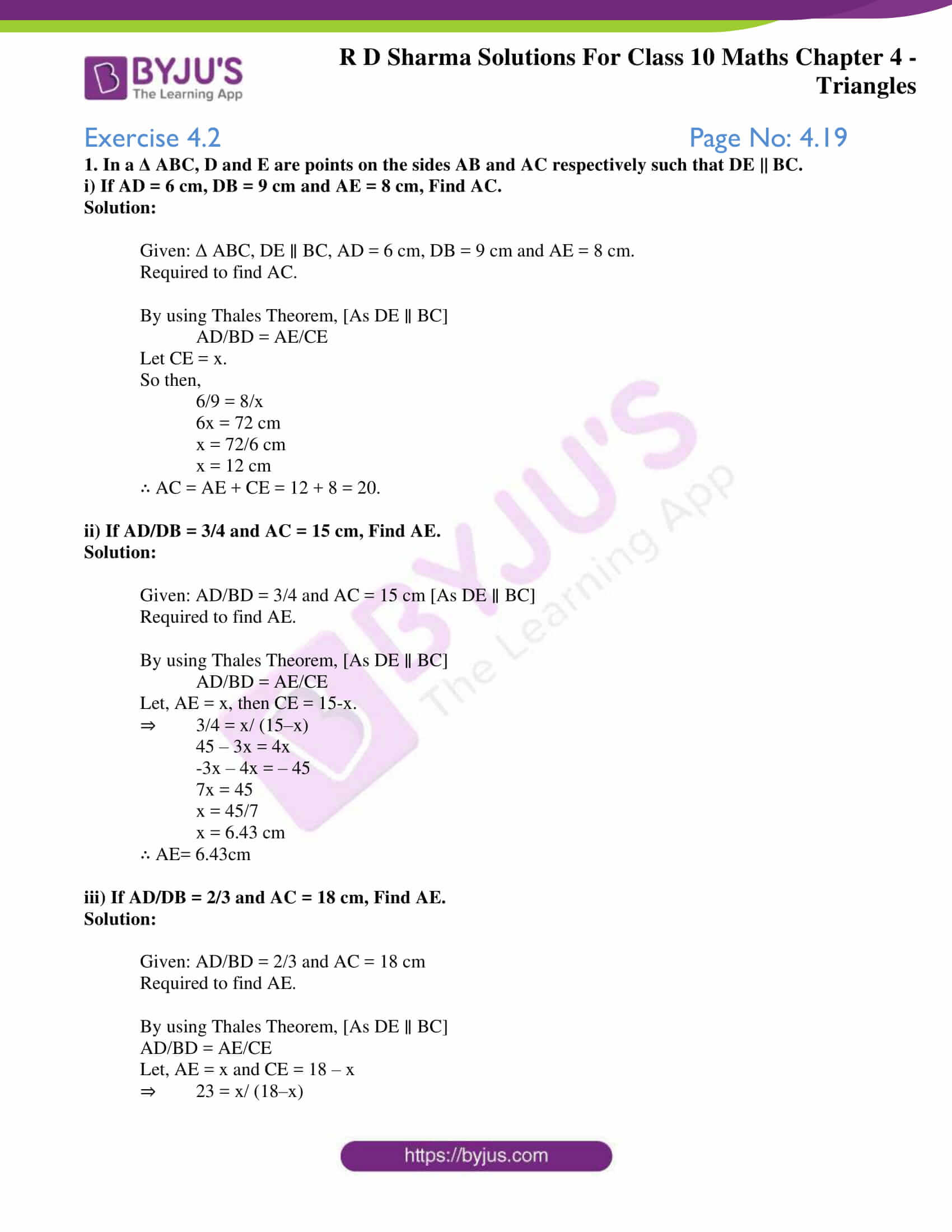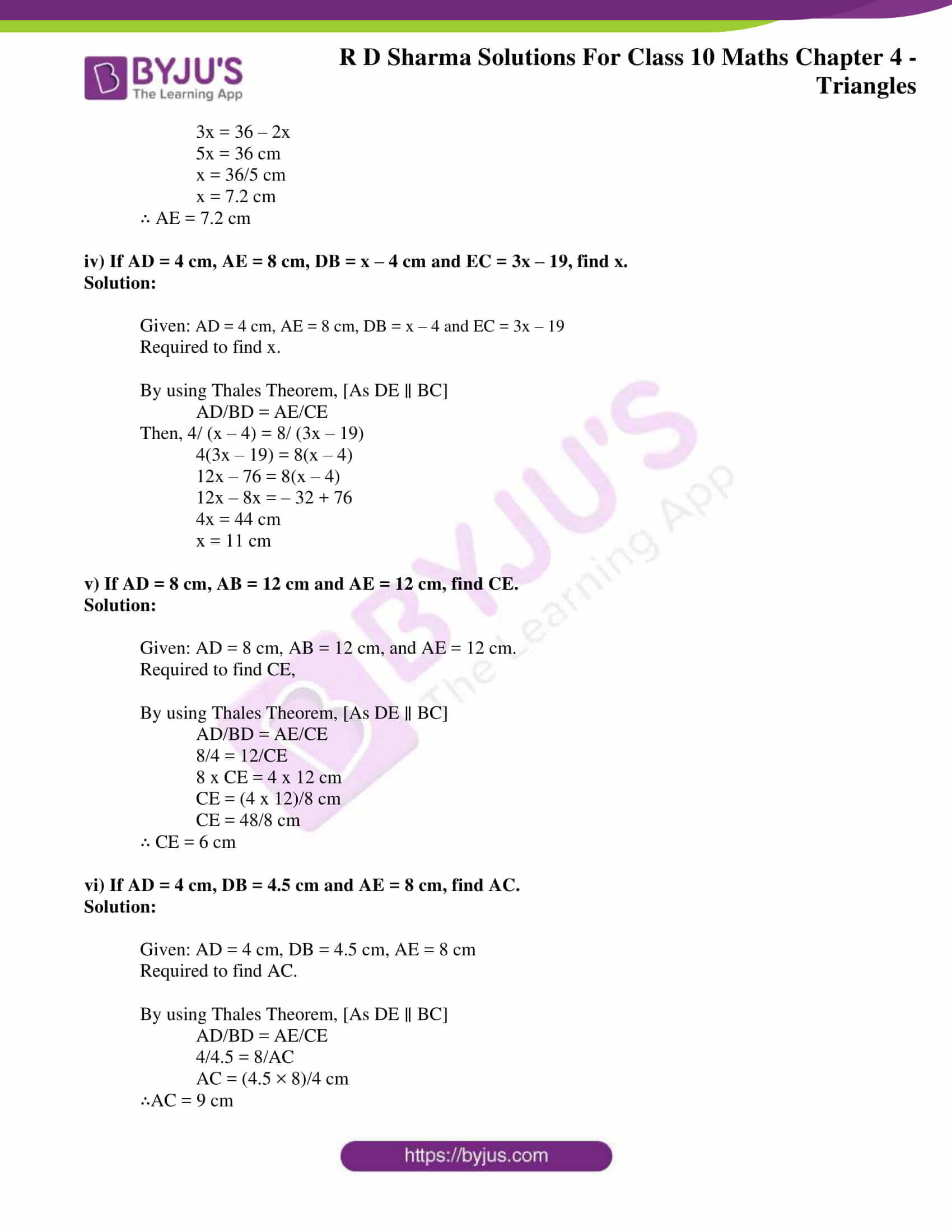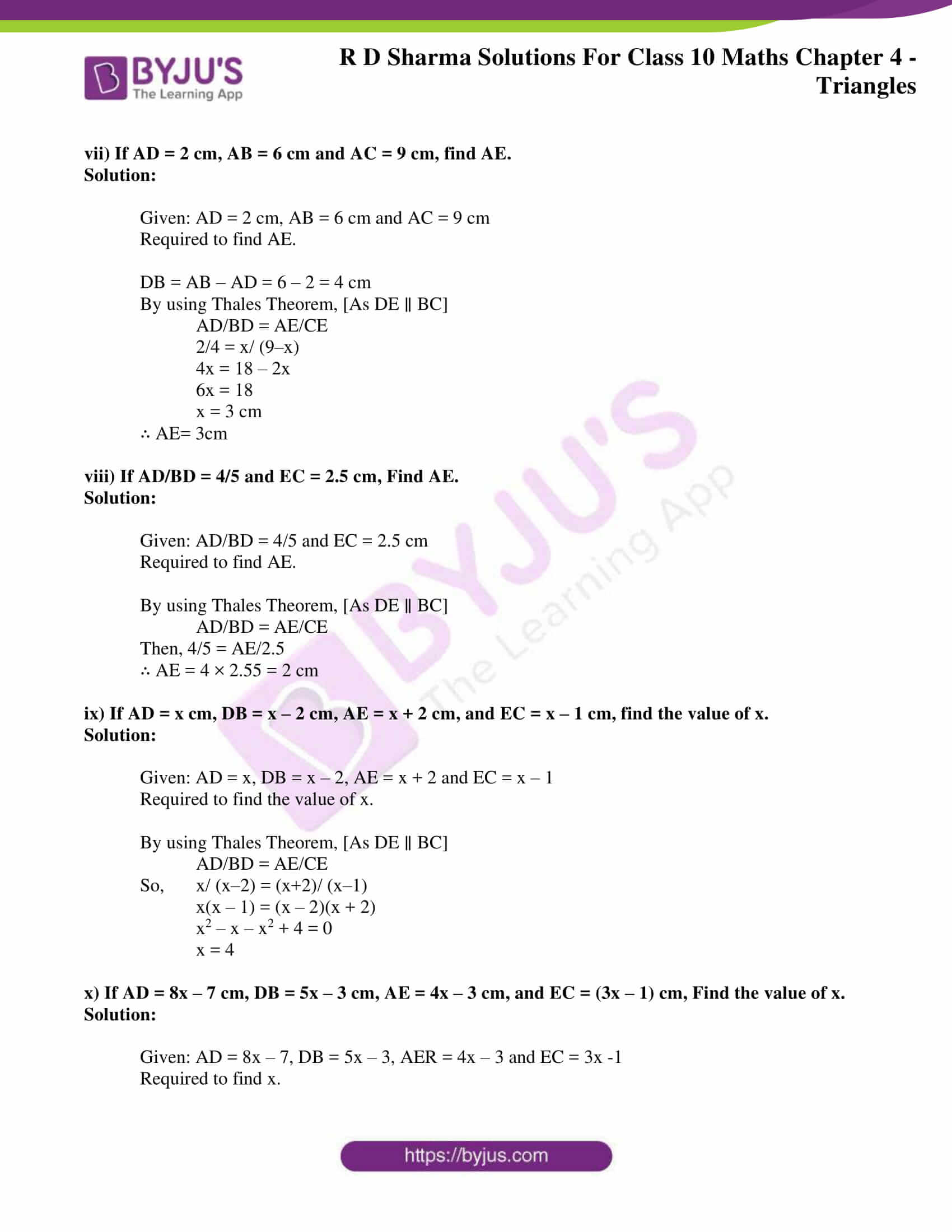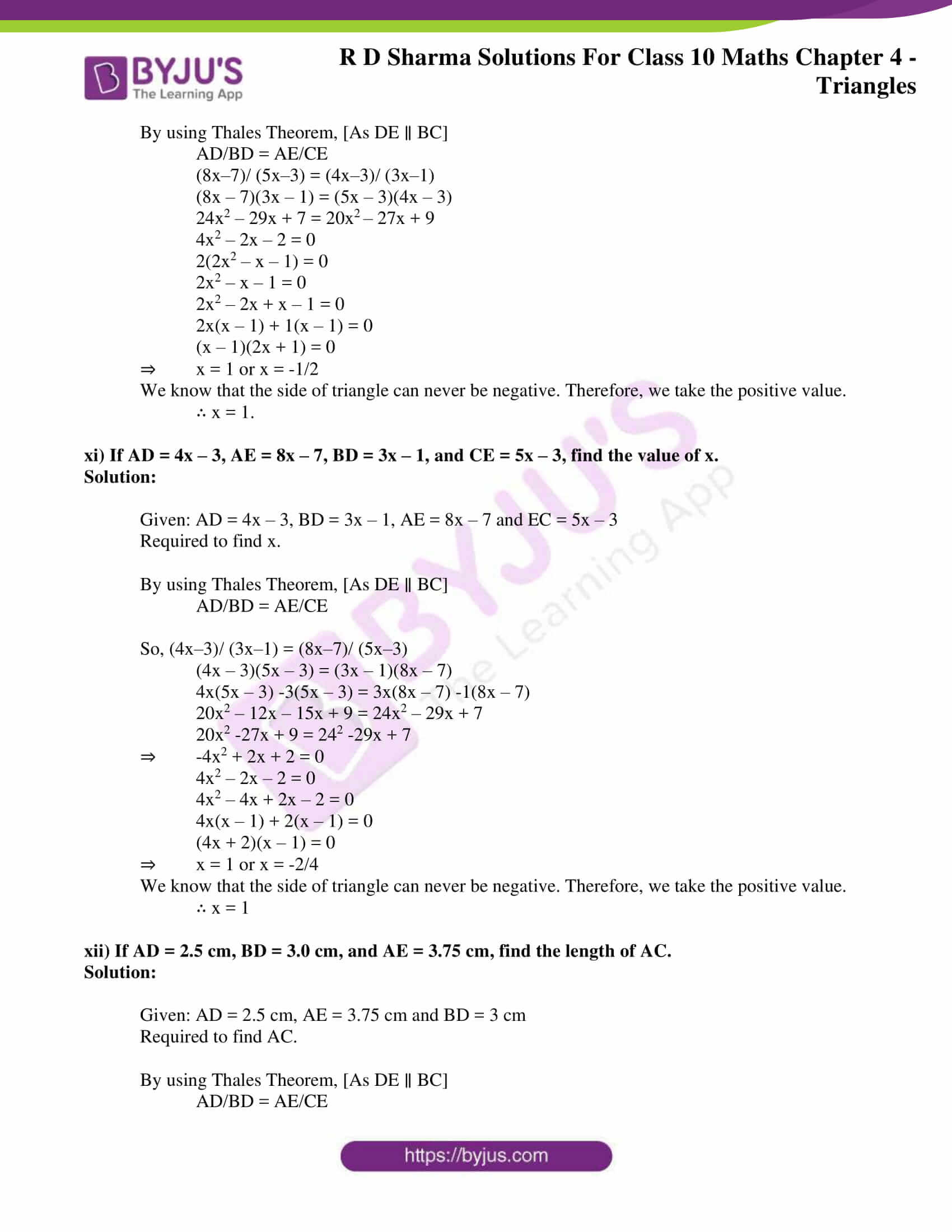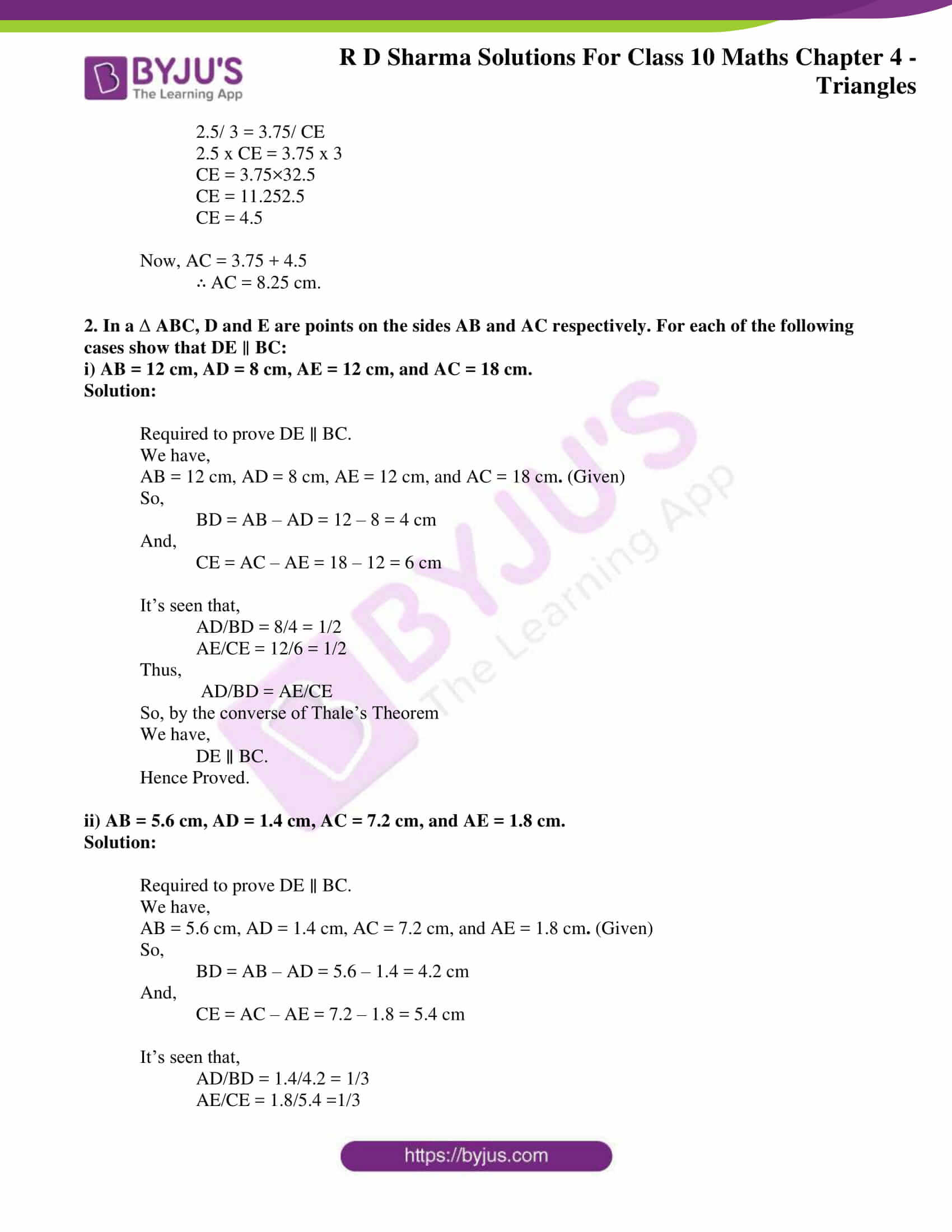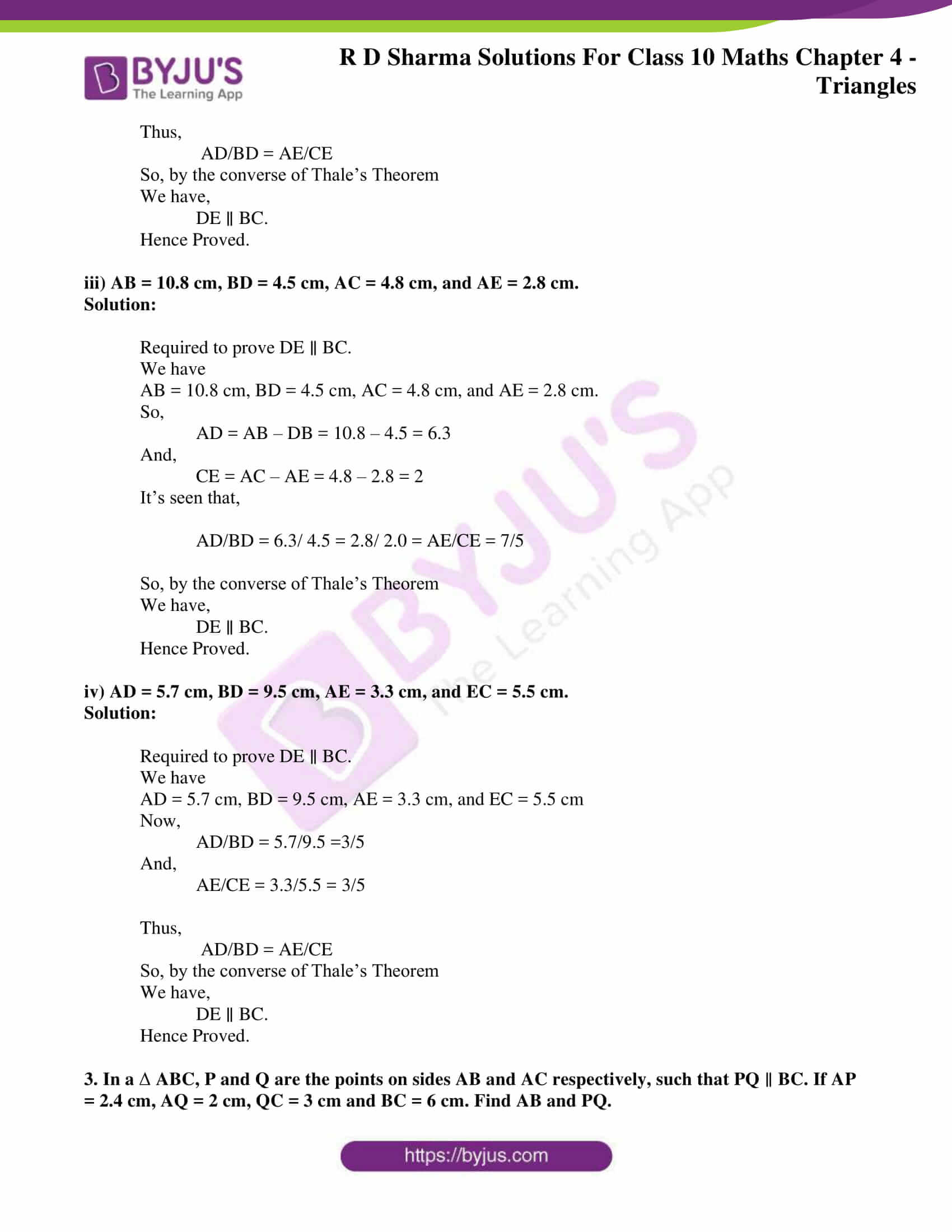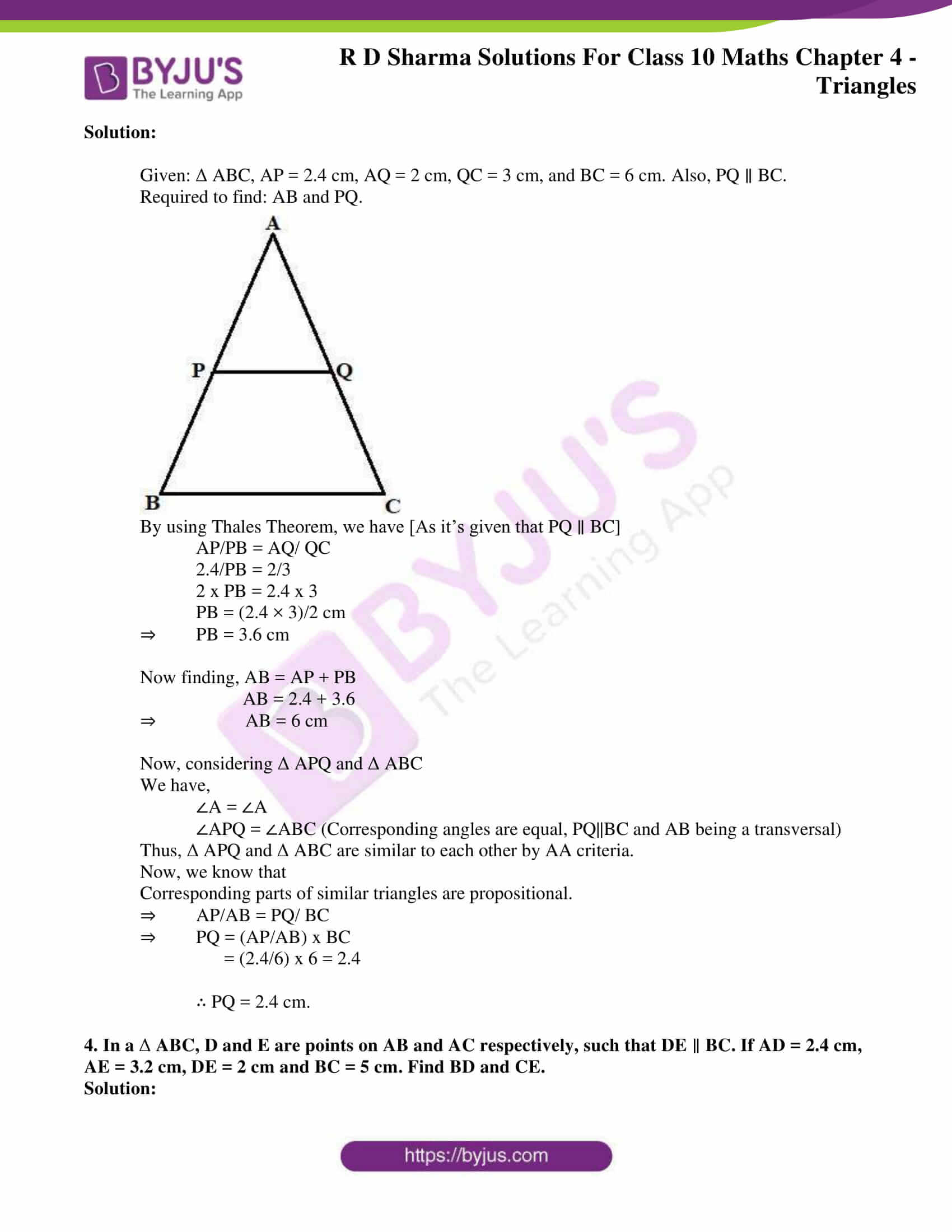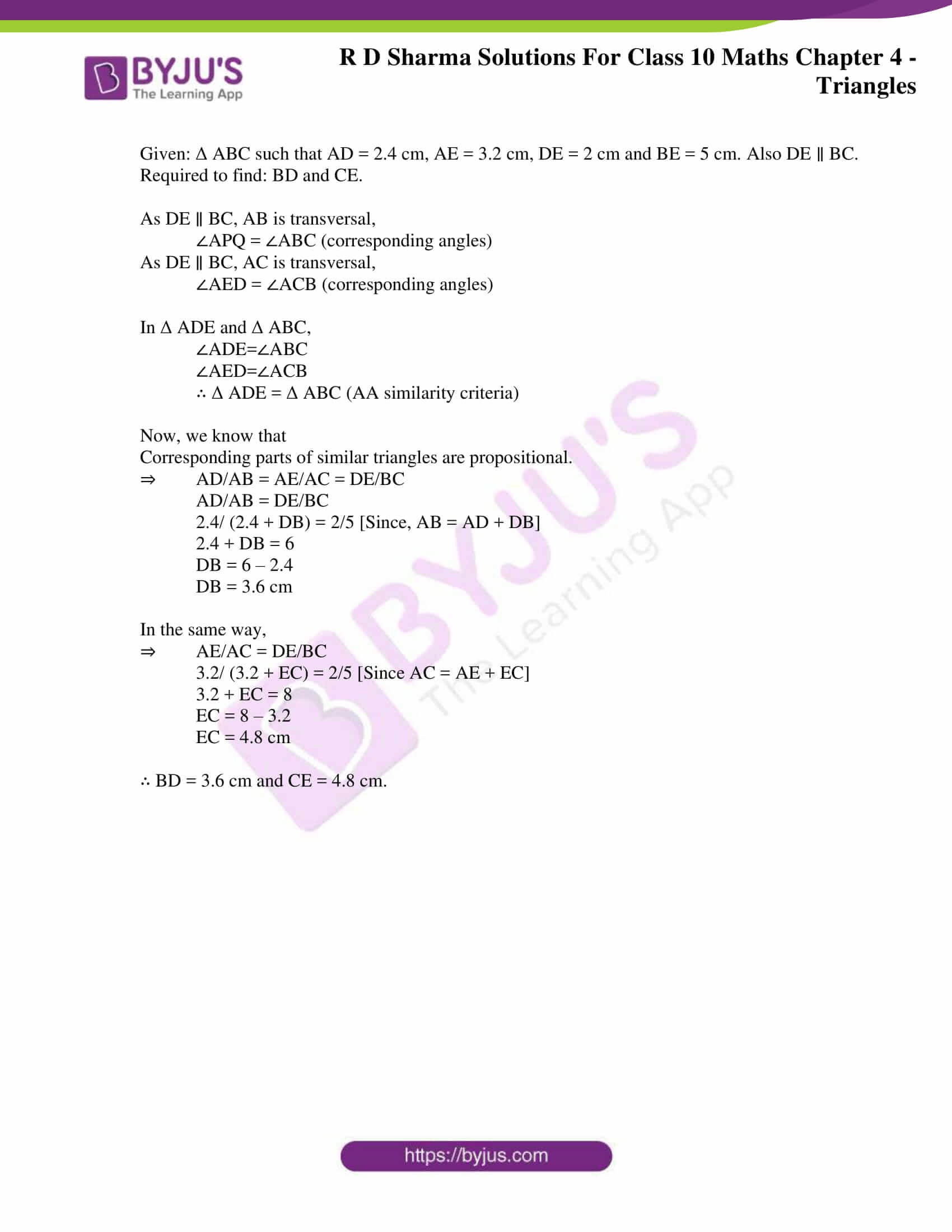### Access RD Sharma Solutions for Class 10 Maths Chapter 4 Triangles Exercise 4.2

1. In a Δ ABC, D and E are points on the sides AB and AC respectively such that DE || BC.

i) If AD = 6 cm, DB = 9 cm and AE = 8 cm, Find AC.

Solution:

Given: Δ ABC, DE ∥ BC, AD = 6 cm, DB = 9 cm and AE = 8 cm.

Required to find AC.

By using Thales Theorem, [As DE ∥ BC]

Let CE = x.

So then,

6/9 = 8/x

6x = 72 cm

x = 72/6 cm

x = 12 cm

∴ AC = AE + CE = 12 + 8 = 20.

ii) If AD/DB = 3/4 and AC = 15 cm, Find AE.

Solution:

Given: AD/BD = 3/4 and AC = 15 cm [As DE ∥ BC]

Required to find AE.

By using Thales Theorem, [As DE ∥ BC]

Let, AE = x, then CE = 15-x.

⇒ 3/4 = x/ (15–x)

45 – 3x = 4x

-3x – 4x = – 45

7x = 45

x = 45/7

x = 6.43 cm

∴ AE= 6.43cm

iii) If AD/DB = 2/3 and AC = 18 cm, Find AE.

Solution:

Given: AD/BD = 2/3 and AC = 18 cm

Required to find AE.

By using Thales Theorem, [As DE ∥ BC]

Let, AE = x and CE = 18 – x

⇒ 23 = x/ (18–x)

3x = 36 – 2x

5x = 36 cm

x = 36/5 cm

x = 7.2 cm

∴ AE = 7.2 cm

iv) If AD = 4 cm, AE = 8 cm, DB = x – 4 cm and EC = 3x – 19, find x.

Solution:

Given: AD = 4 cm, AE = 8 cm, DB = x – 4 and EC = 3x – 19

Required to find x.

By using Thales Theorem, [As DE ∥ BC]

Then, 4/ (x – 4) = 8/ (3x – 19)

4(3x – 19) = 8(x – 4)

12x – 76 = 8(x – 4)

12x – 8x = – 32 + 76

4x = 44 cm

x = 11 cm

v) If AD = 8 cm, AB = 12 cm and AE = 12 cm, find CE.

Solution:

Given: AD = 8 cm, AB = 12 cm, and AE = 12 cm.

Required to find CE,

By using Thales Theorem, [As DE ∥ BC]

8/4 = 12/CE

8 x CE = 4 x 12 cm

CE = (4 x 12)/8 cm

CE = 48/8 cm

∴ CE = 6 cm

vi) If AD = 4 cm, DB = 4.5 cm and AE = 8 cm, find AC.

Solution:

Given: AD = 4 cm, DB = 4.5 cm, AE = 8 cm

Required to find AC.

By using Thales Theorem, [As DE ∥ BC]

4/4.5 = 8/AC

AC = (4.5 × 8)/4 cm

∴AC = 9 cm

vii) If AD = 2 cm, AB = 6 cm and AC = 9 cm, find AE.

Solution:

Given: AD = 2 cm, AB = 6 cm and AC = 9 cm

Required to find AE.

DB = AB – AD = 6 – 2 = 4 cm

By using Thales Theorem, [As DE ∥ BC]

2/4 = x/ (9–x)

4x = 18 – 2x

6x = 18

x = 3 cm

∴ AE= 3cm

viii) If AD/BD = 4/5 and EC = 2.5 cm, Find AE.

Solution:

Given: AD/BD = 4/5 and EC = 2.5 cm

Required to find AE.

By using Thales Theorem, [As DE ∥ BC]

Then, 4/5 = AE/2.5

∴ AE = 4 × 2.55 = 2 cm

ix) If AD = x cm, DB = x – 2 cm, AE = x + 2 cm, and EC = x – 1 cm, find the value of x.

Solution:

Given: AD = x, DB = x – 2, AE = x + 2 and EC = x – 1

Required to find the value of x.

By using Thales Theorem, [As DE ∥ BC]

So, x/ (x–2) = (x+2)/ (x–1)

x(x – 1) = (x – 2)(x + 2)

x2 – x – x2 + 4 = 0

x = 4

x) If AD = 8x – 7 cm, DB = 5x – 3 cm, AE = 4x – 3 cm, and EC = (3x – 1) cm, Find the value of x.

Solution:

Given: AD = 8x – 7, DB = 5x – 3, AER = 4x – 3 and EC = 3x -1

Required to find x.

By using Thales Theorem, [As DE ∥ BC]

(8x–7)/ (5x–3) = (4x–3)/ (3x–1)

(8x – 7)(3x – 1) = (5x – 3)(4x – 3)

24x2 – 29x + 7 = 20x– 27x + 9

4x2 – 2x – 2 = 0

2(2x2 – x – 1) = 0

2x2 – x – 1 = 0

2x2 – 2x + x – 1 = 0

2x(x – 1) + 1(x – 1) = 0

(x – 1)(2x + 1) = 0

⇒ x = 1 or x = -1/2

We know that the side of triangle can never be negative. Therefore, we take the positive value.

∴ x = 1.

xi) If AD = 4x – 3, AE = 8x – 7, BD = 3x – 1, and CE = 5x – 3, find the value of x.

Solution:

Given: AD = 4x – 3, BD = 3x – 1, AE = 8x – 7 and EC = 5x – 3

Required to find x.

By using Thales Theorem, [As DE ∥ BC]

So, (4x–3)/ (3x–1) = (8x–7)/ (5x–3)

(4x – 3)(5x – 3) = (3x – 1)(8x – 7)

4x(5x – 3) -3(5x – 3) = 3x(8x – 7) -1(8x – 7)

20x2 – 12x – 15x + 9 = 24x2 – 29x + 7

20x2 -27x + 9 = 242 -29x + 7

⇒ -4x2 + 2x + 2 = 0

4x2 – 2x – 2 = 0

4x2 – 4x + 2x – 2 = 0

4x(x – 1) + 2(x – 1) = 0

(4x + 2)(x – 1) = 0

⇒ x = 1 or x = -2/4

We know that the side of triangle can never be negative. Therefore, we take the positive value.

∴ x = 1

xii) If AD = 2.5 cm, BD = 3.0 cm, and AE = 3.75 cm, find the length of AC.

Solution:

Given: AD = 2.5 cm, AE = 3.75 cm and BD = 3 cm

Required to find AC.

By using Thales Theorem, [As DE ∥ BC]

2.5/ 3 = 3.75/ CE

2.5 x CE = 3.75 x 3

CE = 3.75×32.5

CE = 11.252.5

CE = 4.5

Now, AC = 3.75 + 4.5

∴ AC = 8.25 cm.

2. In a Δ ABC, D and E are points on the sides AB and AC respectively. For each of the following cases show that DE  BC:

i) AB = 12 cm, AD = 8 cm, AE = 12 cm, and AC = 18 cm.

Solution:

Required to prove DE ∥ BC.

We have,

AB = 12 cm, AD = 8 cm, AE = 12 cm, and AC = 18 cm. (Given)

So,

BD = AB – AD = 12 – 8 = 4 cm

And,

CE = AC – AE = 18 – 12 = 6 cm

It’s seen that,

AE/CE = 12/6 = 1/2

Thus,

So, by the converse of Thale’s Theorem

We have,

DE ∥ BC.

Hence Proved.

ii) AB = 5.6 cm, AD = 1.4 cm, AC = 7.2 cm, and AE = 1.8 cm.

Solution:

Required to prove DE ∥ BC.

We have,

AB = 5.6 cm, AD = 1.4 cm, AC = 7.2 cm, and AE = 1.8 cm. (Given)

So,

BD = AB – AD = 5.6 – 1.4 = 4.2 cm

And,

CE = AC – AE = 7.2 – 1.8 = 5.4 cm

It’s seen that,

AE/CE = 1.8/5.4 =1/3

Thus,

So, by the converse of Thale’s Theorem

We have,

DE ∥ BC.

Hence Proved.

iii) AB = 10.8 cm, BD = 4.5 cm, AC = 4.8 cm, and AE = 2.8 cm.

Solution:

Required to prove DE ∥ BC.

We have

AB = 10.8 cm, BD = 4.5 cm, AC = 4.8 cm, and AE = 2.8 cm.

So,

AD = AB – DB = 10.8 – 4.5 = 6.3

And,

CE = AC – AE = 4.8 – 2.8 = 2

It’s seen that,

AD/BD = 6.3/ 4.5 = 2.8/ 2.0 = AE/CE = 7/5

So, by the converse of Thale’s Theorem

We have,

DE ∥ BC.

Hence Proved.

iv) AD = 5.7 cm, BD = 9.5 cm, AE = 3.3 cm, and EC = 5.5 cm.

Solution:

Required to prove DE ∥ BC.

We have

AD = 5.7 cm, BD = 9.5 cm, AE = 3.3 cm, and EC = 5.5 cm

Now,

And,

AE/CE = 3.3/5.5 = 3/5

Thus,

So, by the converse of Thale’s Theorem

We have,

DE ∥ BC.

Hence Proved.

3. In a Δ ABC, P and Q are the points on sides AB and AC respectively, such that PQ  BC. If AP = 2.4 cm, AQ = 2 cm, QC = 3 cm and BC = 6 cm. Find AB and PQ.

Solution:

Given: Δ ABC, AP = 2.4 cm, AQ = 2 cm, QC = 3 cm, and BC = 6 cm. Also, PQ ∥ BC.

Required to find: AB and PQ.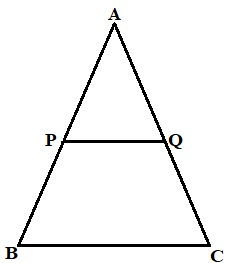By using Thales Theorem, we have [As it’s given that PQ ∥ BC]

AP/PB = AQ/ QC

2.4/PB = 2/3

2 x PB = 2.4 x 3

PB = (2.4 × 3)/2 cm

⇒ PB = 3.6 cm

Now finding, AB = AP + PB

AB = 2.4 + 3.6

⇒ AB = 6 cm

Now, considering Δ APQ and Δ ABC

We have,

∠A = ∠A

∠APQ = ∠ABC (Corresponding angles are equal, PQ||BC and AB being a transversal)

Thus, Δ APQ and Δ ABC are similar to each other by AA criteria.

Now, we know that

Corresponding parts of similar triangles are propositional.

⇒ AP/AB = PQ/ BC

⇒ PQ = (AP/AB) x BC

= (2.4/6) x 6 = 2.4

∴ PQ = 2.4 cm.

4. In a Δ ABC, D and E are points on AB and AC respectively, such that DE  BC. If AD = 2.4 cm, AE = 3.2 cm, DE = 2 cm and BC = 5 cm. Find BD and CE.

Solution:

Given: Δ ABC such that AD = 2.4 cm, AE = 3.2 cm, DE = 2 cm and BE = 5 cm. Also DE ∥ BC.

Required to find: BD and CE.

As DE ∥ BC, AB is transversal,

∠APQ = ∠ABC (corresponding angles)

As DE ∥ BC, AC is transversal,

∠AED = ∠ACB (corresponding angles)

In Δ ADE and Δ ABC,

∠AED=∠ACB

∴ Δ ADE = Δ ABC (AA similarity criteria)

Now, we know that

Corresponding parts of similar triangles are propositional.

⇒ AD/AB = AE/AC = DE/BC

2.4/ (2.4 + DB) = 2/5 [Since, AB = AD + DB]

2.4 + DB = 6

DB = 6 – 2.4

DB = 3.6 cm

In the same way,

⇒ AE/AC = DE/BC

3.2/ (3.2 + EC) = 2/5 [Since AC = AE + EC]

3.2 + EC = 8

EC = 8 – 3.2

EC = 4.8 cm

∴ BD = 3.6 cm and CE = 4.8 cm.